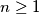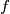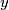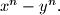### IMO Shortlist 2011 problem N3

Kvaliteta:
Avg: 4.0
Težina:
Avg: 6.5
Let$n \geq 1$ be an odd integer. Determine all functions$f$ from the set of integers to itself, such that for all integers$x$ and$y$ the difference$f(x)-f(y)$ divides$x^n-y^n.$

Proposed by Mihai Baluna, Romania
Source: Međunarodna matematička olimpijada, shortlist 2011